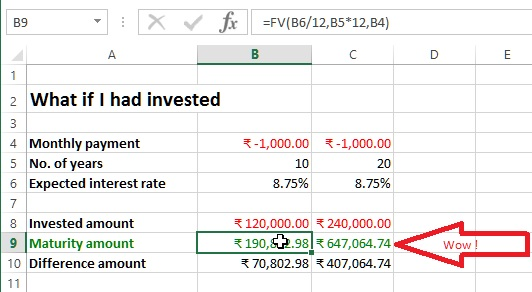# What if I had invested calculation in Excel

##### How to do what if I had invested calculations in MS Excel?

In previous post, we learnt about Calculate monthly installment of a loan in Excel. In this post, we shall learn 'What if I had invested scenario' in Excel. We will learn what If I would have invested a certain amount for certain period assuming to get certain interest rate then how much amount we would end up getting.

Before we go ahead with this calculations, we need to understand the behavior of Excel.

#### Important: How excel treat an amount

Excel treat all payment as negative amount and all receipt as positive amount. So when we are paying monthly installment, its negative amount in the formula.

### What if I had invested

To get the maturity amount again, we shall use FV function of Excel. Look at below scenario, where we are assuming that I would

• invest 1000 a month (this should be negative amount as we are paying)
• for 10 years
• and assuming I will get 8.75% interest per year
In B9, we have entered formula '=PV(B6/12,B5*12,B4)' where

• 1st parameter is B6/12 that is interest rate (B6/12 as 8.75% is the yearly rate of interest)
• 2nd parameter is B5*12 that is term in year (B5*12 as term is in year and installment is monthly)
• 3rd parameter is B4 is monthly payment
and the result is 190,802.98.
Total amount invested is '12*10*1000=120,000' and you end up gaining '79,791.42'.Annuity investment

Let's say that we want to buy an annuity plan that will pay us 1000 every month for next 10 years at the rate of 8.75%, how much we need to invest?

To calculate the annuity amount, enter '=PV(B6/12,B5*12,B4)' formula in B12, here

• 1st parameter is B6/12 that is interest rate per month
• 2nd parameter is B5*12 that receipt per month
• 3rd parameter is B4 that is payment per month

and the result is 79,791.42.Thanks for reading, if this helps do share to your friends and colleagues.

Views: 2359 | Post Order: 34